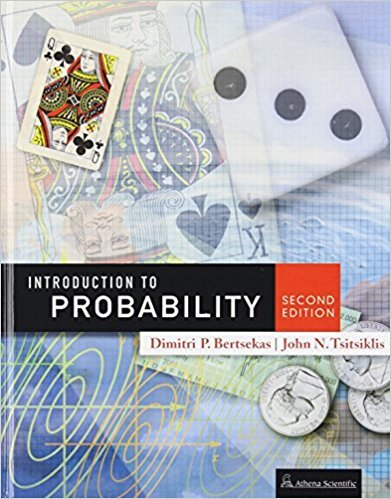×
×

# Solutions for Chapter 2: Discrete Random Variables## Full solutions for Introduction to Probability, | 2nd Edition

ISBN: 9781886529236Solutions for Chapter 2: Discrete Random Variables

Solutions for Chapter 2
4 5 0 395 Reviews
14
4
##### ISBN: 9781886529236

Chapter 2: Discrete Random Variables includes 46 full step-by-step solutions. This textbook survival guide was created for the textbook: Introduction to Probability,, edition: 2. Introduction to Probability, was written by and is associated to the ISBN: 9781886529236. Since 46 problems in chapter 2: Discrete Random Variables have been answered, more than 7418 students have viewed full step-by-step solutions from this chapter. This expansive textbook survival guide covers the following chapters and their solutions.

Key Statistics Terms and definitions covered in this textbook

A formula used to determine the probability of the union of two (or more) events from the probabilities of the events and their intersection(s).

• Arithmetic mean

The arithmetic mean of a set of numbers x1 , x2 ,…, xn is their sum divided by the number of observations, or ( / )1 1 n xi t n ? = . The arithmetic mean is usually denoted by x , and is often called the average

• Attribute control chart

Any control chart for a discrete random variable. See Variables control chart.

• Axioms of probability

A set of rules that probabilities deined on a sample space must follow. See Probability

• Bimodal distribution.

A distribution with two modes

• Binomial random variable

A discrete random variable that equals the number of successes in a ixed number of Bernoulli trials.

• Bivariate normal distribution

The joint distribution of two normal random variables

• Cause-and-effect diagram

A chart used to organize the various potential causes of a problem. Also called a ishbone diagram.

• Combination.

A subset selected without replacement from a set used to determine the number of outcomes in events and sample spaces.

• Continuous distribution

A probability distribution for a continuous random variable.

• Correlation coeficient

A dimensionless measure of the linear association between two variables, usually lying in the interval from ?1 to +1, with zero indicating the absence of correlation (but not necessarily the independence of the two variables).

• Critical value(s)

The value of a statistic corresponding to a stated signiicance level as determined from the sampling distribution. For example, if PZ z PZ ( )( .) . ? =? = 0 025 . 1 96 0 025, then z0 025 . = 1 9. 6 is the critical value of z at the 0.025 level of signiicance. Crossed factors. Another name for factors that are arranged in a factorial experiment.

• Density function

Another name for a probability density function

• Eficiency

A concept in parameter estimation that uses the variances of different estimators; essentially, an estimator is more eficient than another estimator if it has smaller variance. When estimators are biased, the concept requires modiication.

• Error propagation

An analysis of how the variance of the random variable that represents that output of a system depends on the variances of the inputs. A formula exists when the output is a linear function of the inputs and the formula is simpliied if the inputs are assumed to be independent.

• Error sum of squares

In analysis of variance, this is the portion of total variability that is due to the random component in the data. It is usually based on replication of observations at certain treatment combinations in the experiment. It is sometimes called the residual sum of squares, although this is really a better term to use only when the sum of squares is based on the remnants of a model-itting process and not on replication.

• Event

A subset of a sample space.

• Experiment

A series of tests in which changes are made to the system under study

• Extra sum of squares method

A method used in regression analysis to conduct a hypothesis test for the additional contribution of one or more variables to a model.

• F-test

Any test of signiicance involving the F distribution. The most common F-tests are (1) testing hypotheses about the variances or standard deviations of two independent normal distributions, (2) testing hypotheses about treatment means or variance components in the analysis of variance, and (3) testing signiicance of regression or tests on subsets of parameters in a regression model.

×